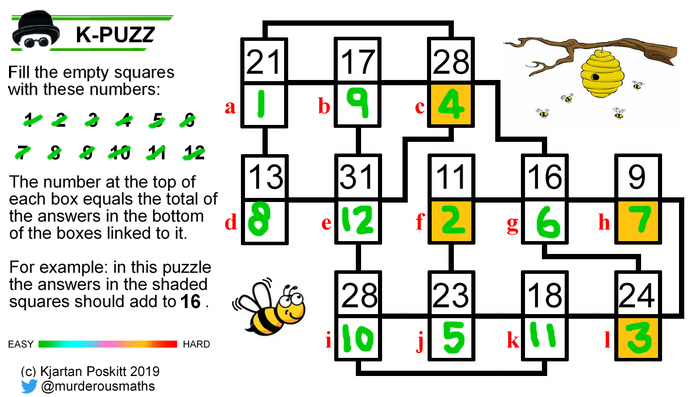#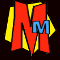The K-PUZZ Logic Puzzles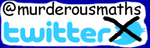K-PUZZ logic puzzles have appeared regularily on the Murderous Maths Twitter Page since May 2019. They are based on the original Kjarposko Puzzles which are usually larger and more complex. Teachers are now using these puzzles in class for some fun practice with numbers and logic!

## How do they work?

The video introduces the puzzles and shows a couple being solved.

There are also step-by-step guides below. The red letters are for these explanations only. Numbers in [brackets] refer to the clues in the puzzle.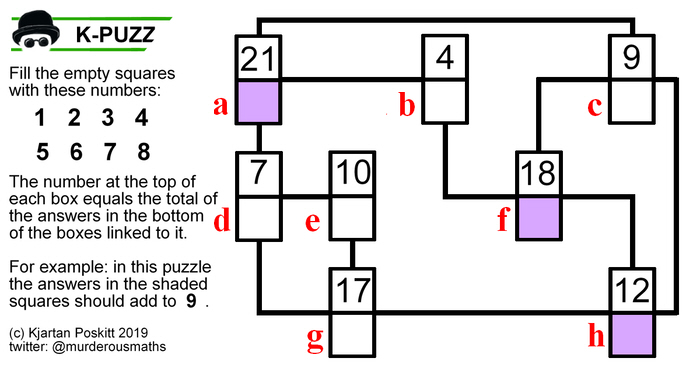In this puzzle, the answers a + f + h must add to . As a + f = , therefore h =  -  = 5 As b + c + h =  and we now know h = 5, therefore b + c =  - 5 = 13. Hold that thought. b + c + d = , but remember that b + c = 13, so d =  - 13 = 8 As d + g = , therefore g =  - 8 = 2 Let's see where we've got to...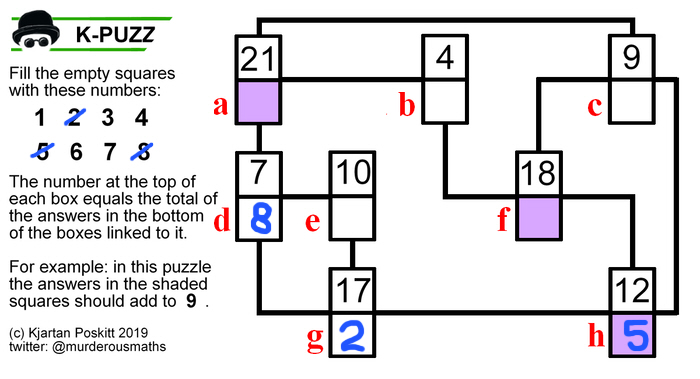Another way to start this puzzle is to look at  and .  can only be made by adding 1 + 2 + 4 and  can only be made by adding 1 + 3. Therefore the 1 must go in the box which is common to both of them which is a. To complete , e = 4 and to complete , f = 3. To complete , c = 7 and finally b = 6. Here's the finished puzzle: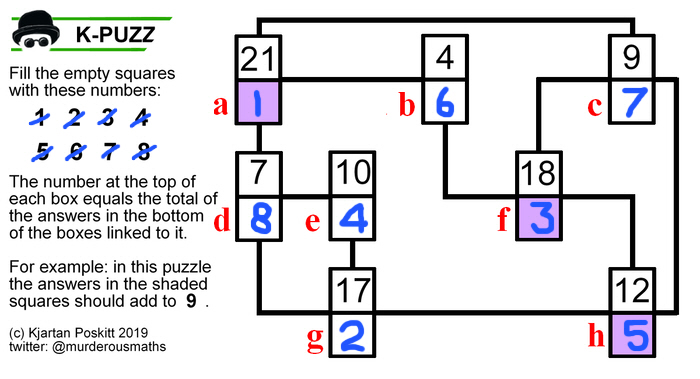You can see a harder example below.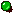Try ourDAILY KJARPOSKO PUZZLE!

## Things to look out for...

### A Loop Of Four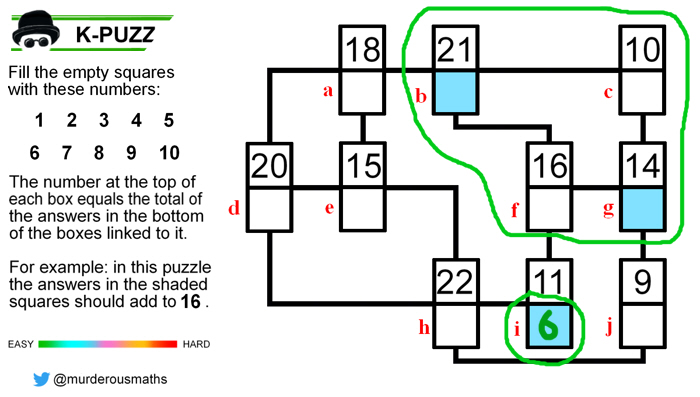In this puzzle, answers b, c, f and g are linked in a loop of four, and  is only linked to two other boxes. This means b and g add to , so to complete , i must = 6.

### A Split (or Shared Pair)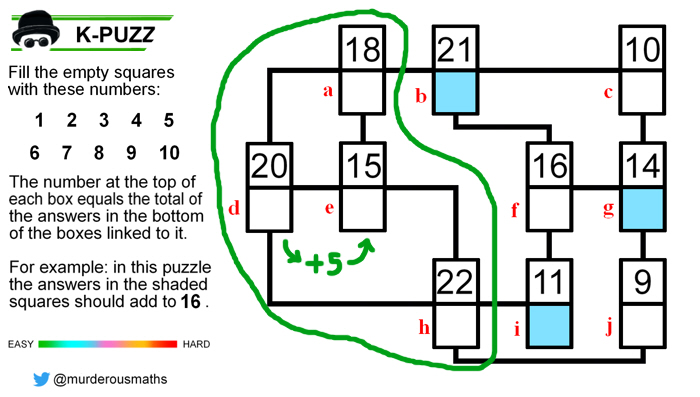Here, answers d and e both link to a and h as well as each other. As  is 5 more than , therefore e must be 5 more than d.

### The Total Count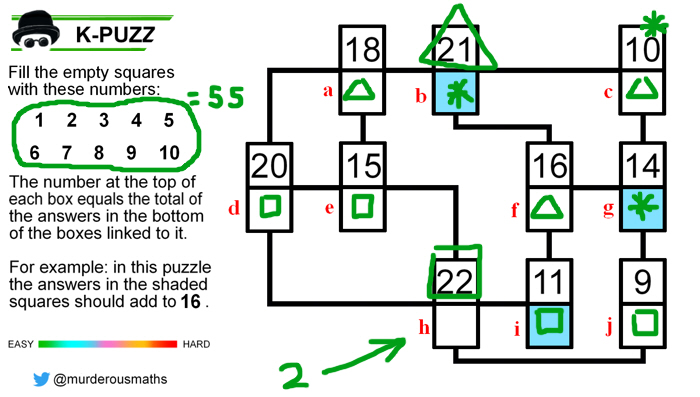Getting the first answer in place is usually the hardest job, but sometimes there's a cheeky way to do it.

In this puzzle the total of all the ten answers = 1+2+3+...+ 10 = 55. Look at how the numbers are linked. ,  and  connect to nine different answers, shown here with triangles, stars and squares. The total of these nine answers is 21 +10 +22 = 53. The only answer that is not used is h therefore h must be 55-53 = 2.

This trick isn't always possible, but if you're stuck it's worth trying to find!

## K-PUZZ variations

The puzzles can appear in a variety of shapes and formats, including novelty images.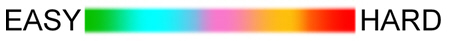K-PUZZ can vary from EASY to HARD, indicated by the colour of the shaded squares. As a guide, the target time for an easy puzzle is 1 minute, and for a hard puzzle 5 minutes.

Some of the harder puzzles don't give all the clues, instead the numbers are replaced by an [*]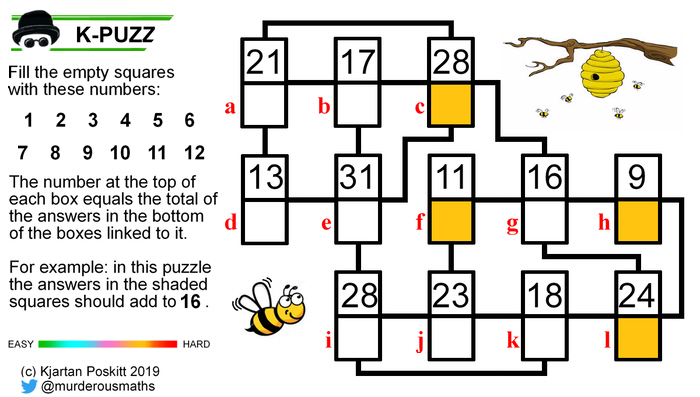a + e =  therefore c =  - 13 = 4 d + b =  - 4 = 17 so the  tells us i = 31-17-4 = 10. j + l =  - 10 = 8. Also g + l = . Therefore g = j +1. However g + j =  therefore g = 6 and j = 5. l =  - 6 = 3. Let's see where we've got to...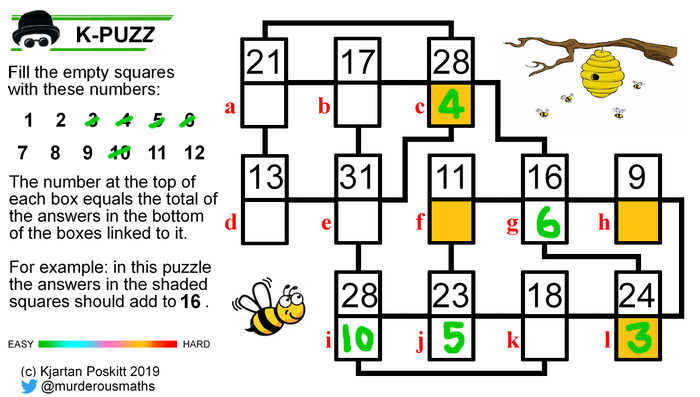The upper  = a + e + b + 6 but as = a + e = , therefore b =  - 13 - 6 = 9. To complete  d = 8 To complete  k + h = 18. The only options left are 11+7. If k = 7 then to complete  we need a 6, but it's already been used. Therefore k = 11 and h = 7. To complete  f = 2. To complete the lower  e = 12. To complete  a = 1. Here's the finished puzzle: